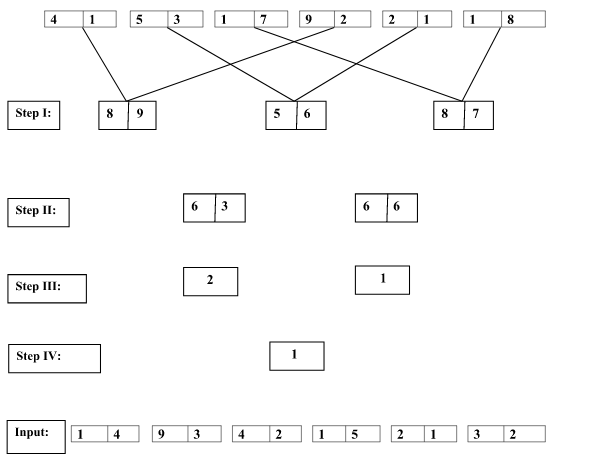# Crack IBPS Clerk Mains– Logical Reasoning (New pattern Input Output)

## Crack IBPS Clerk Mains– Logical Reasoning (New pattern Input Output):

Dear Readers, IBPS is conducting Online Examination for the recruitment of Clerical Cadre. Main Examination of  IBPS Clerk was scheduled from 21st Jan 2018. To enrich your preparation here we have providing new series of Practice Questions on Reasoning Ability. Candidates those who are appearing in IBPS Clerk Exam can practice these questions daily and make your preparation effective.

[WpProQuiz 895]

### Click “Start Quiz” to attend these Questions and view Solutions

Directions (Q. 1-5): Study the following information carefully and answer the given questions.

A number arrangement machine arranges two digit numbers in typical manner.  The first step has been obtained by multiplying the digits in input.Multiplication has not been done in any other steps. They are obtained by applying certain logic. Each step is a resultant of previous step.1)If each digit in the step I is added and then halved, then what will be the final sum?

1. 18
2. 17
3. 19
4. 17.5
5. 18.5

2)Which of the following combination represent the first digit of the second number and second digit of the third number in step I of the given input?

1. (6,8)
2. (9,6)
3. (9,8)
4. (6,9)
5. None of these

3)Which of the following represent the difference between the first digit of the second number and second digit of the first number in step II?

1. -2
2. 2
3. 3
4. -3
5. None of these

4)What is the multiplication of two numbers obtained in step III?

1. 0
2. 1.5
3. 1
4. 2
5. None of these

5)If the value ‘1.35’ is subtracted from the final output, then what will be the resultant value?

1. 1
2. -0.5
3. -1.25
4. -0.85
5. None of these

Directions (Q.6-10): Study the following information carefully and answer the given questions.

A number sorting machine when given an input of numbers, rearranges the numbers in a particular rule in each step. The following is an illustration of input and rearrangement. (Note: All the given input numbers are two digit numbers)

Input: 48  37  29  52  33  45

Step-I: 32  21  18  10  9  20

Step-II: 11  3  8  1  11

Step-III: 2  3  8  1  2

Step-IV: 5  11  9  3

Step-V: 5  2  9  3

Step-VI: 3  7  6

Step-VII: 10  13

Step-VIII: 1 4

Step-IX: 3

Step-IX is the last step of the above arrangement as the intended arrangement is obtained.

As per the rules followed in the given steps, find out the appropriate steps for the given input.

Input: 84  35  41  28  36  72

6)What is the difference between the highest number in step-IV and the lowest number in step-II?

1. 6
2. 8
3. 5
4. 4
5. None of these

7)What is the average value of step-III of given input?

1. 4.2
2. 5.2
3. 3.8
4. 3.6
5. None of these

8)Which of the following number is second from the right end in step-V?

1. 5
2. 6
3. 4
4. 2
5. None of these

9)Which of the following is the penultimate step?

1. 6  10  11
2. 4  0  1
3. 4  1 2
4. 4  1
5. None of these

10)What will be the definite input for the below given step-II, if all the input two digit numbers are below 30?

Step-II: 4  5  14  3  9 1

1. 22  15  27  13  19  11
2. 14  15  27  13  33  11
3. 22  15  27  13  33  10
4. Cannot be determined
5. None of these